Ex 14.2

Chapter 14 Class 10 Statistics (Term 2)
Serial order wise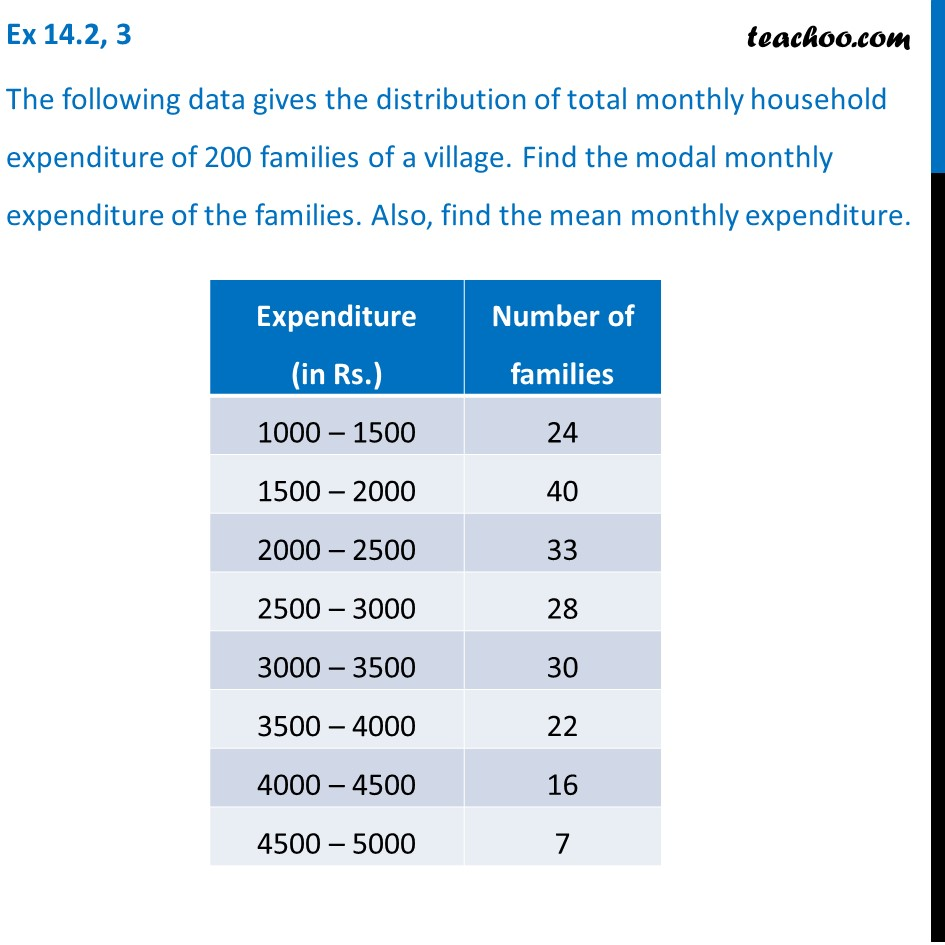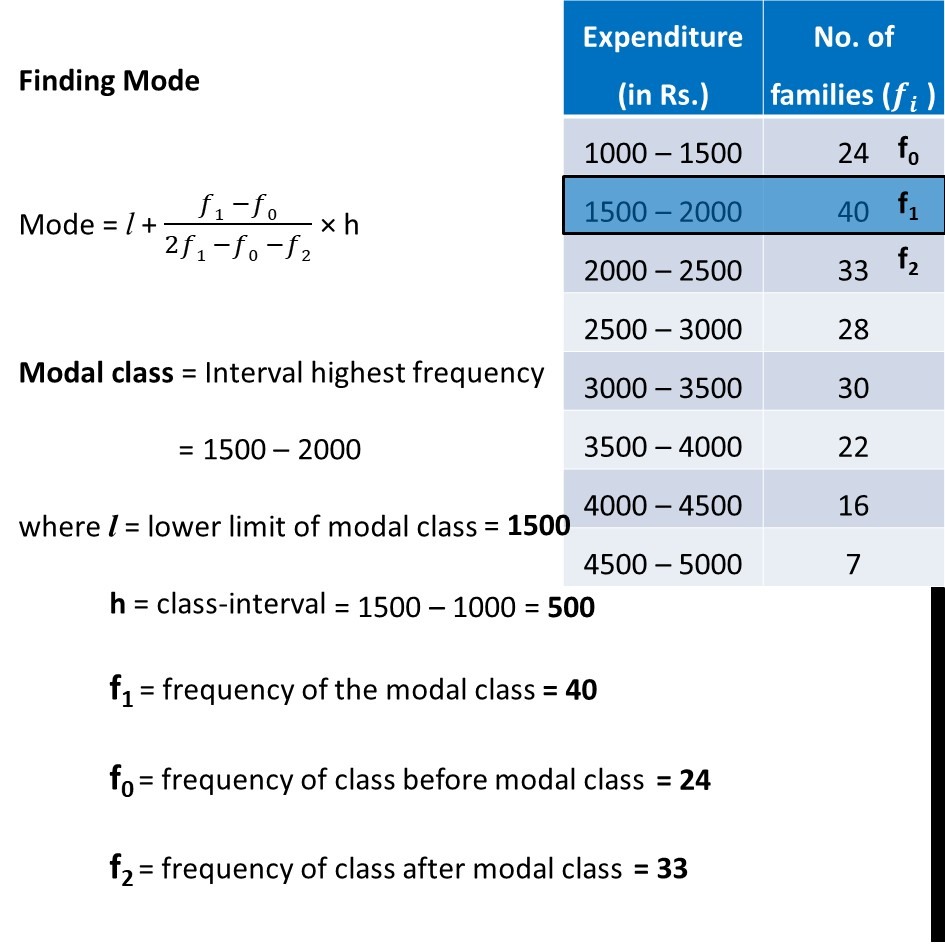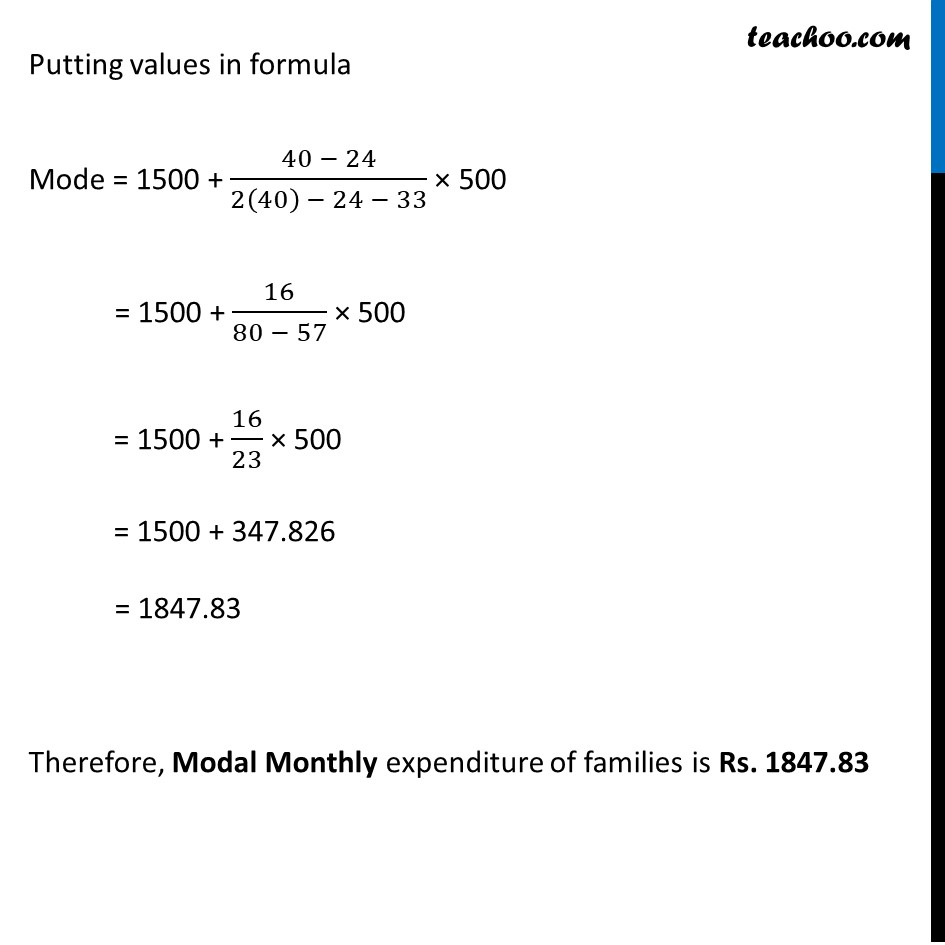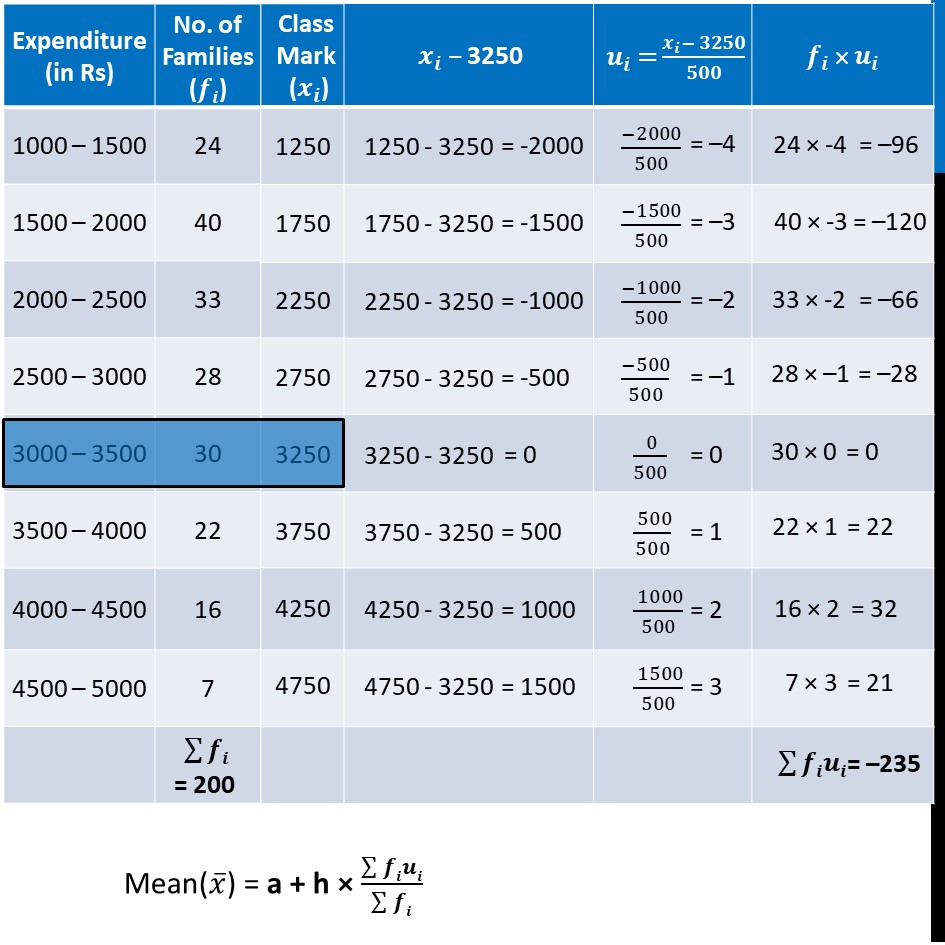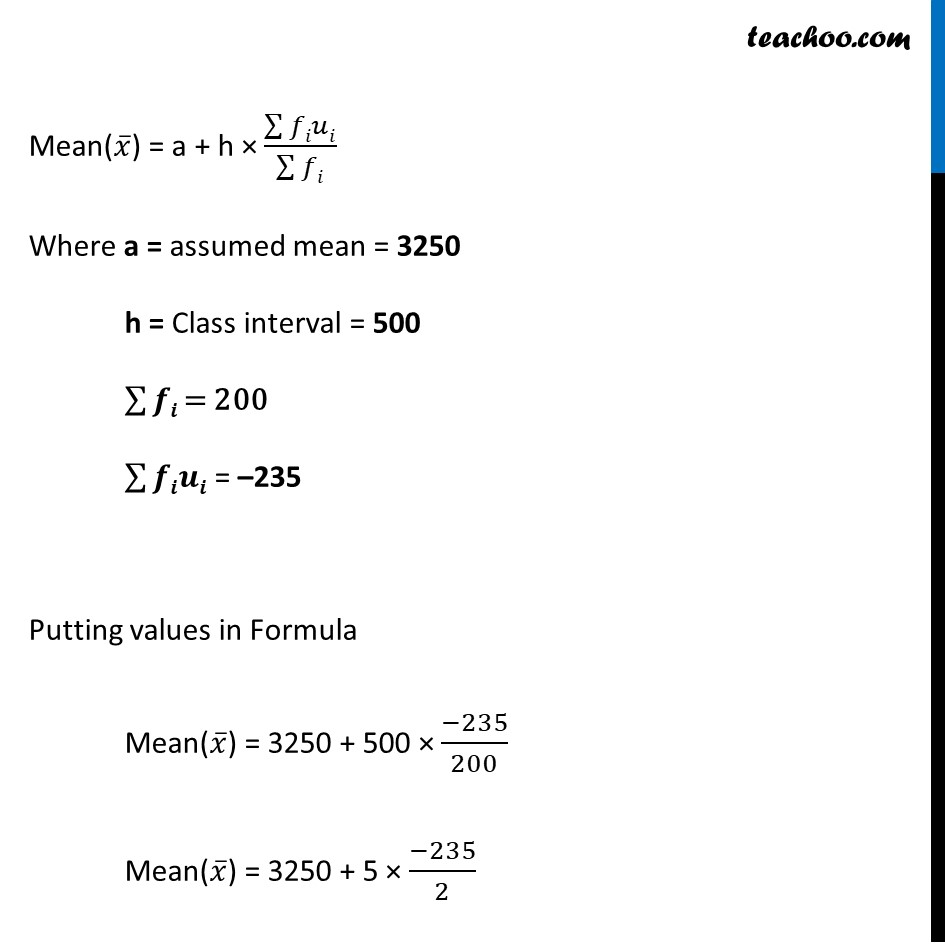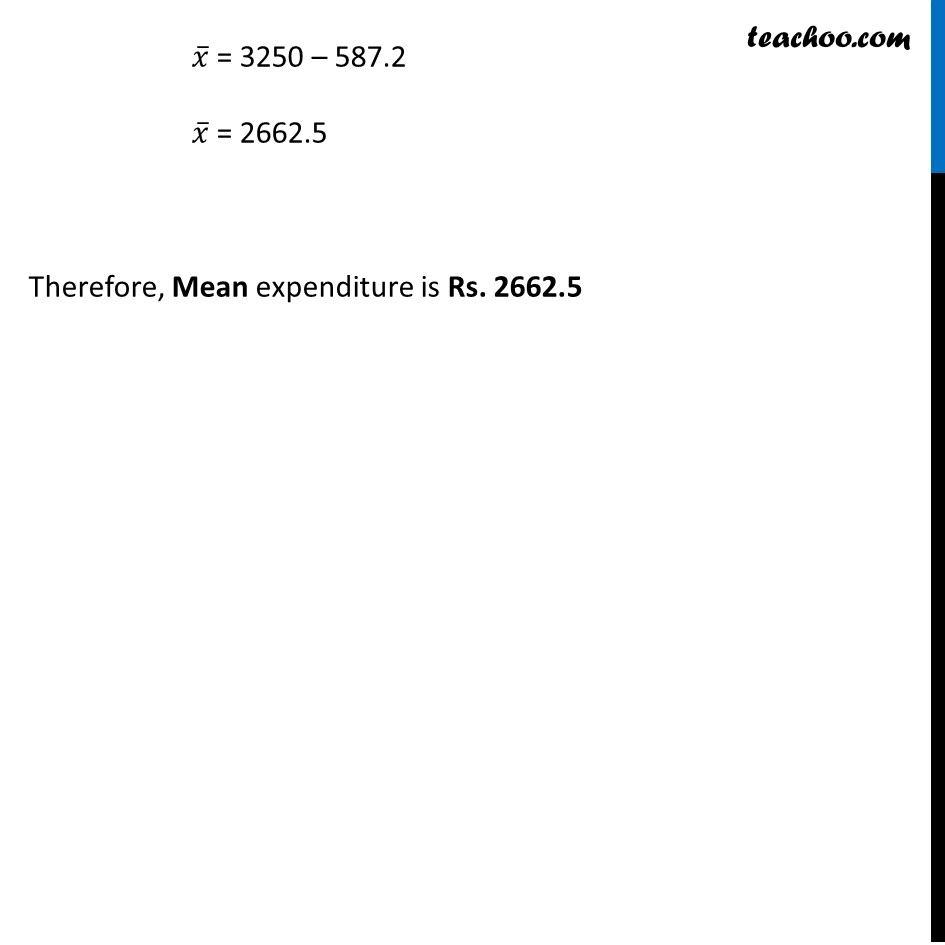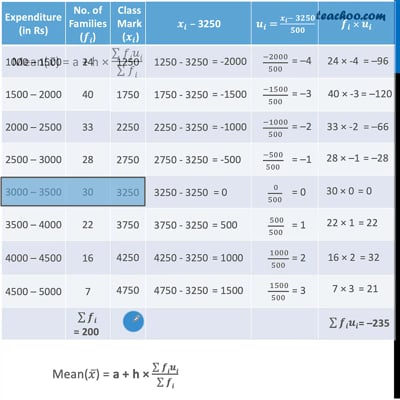This video is only available for Teachoo black users

### Transcript

Ex 14.2, 3 The following data gives the distribution of total monthly household expenditure of 200 families of a village. Find the modal monthly expenditure of the families. Also, find the mean monthly expenditure. Finding Mode Mode = l + (𝑓1 −𝑓0)/(2𝑓1 −𝑓0 −𝑓2) × h Modal class = Interval highest frequency = 1500 – 2000 where l = lower limit of modal class h = class-interval f1 = frequency of the modal class f0 = frequency of class before modal class f2 = frequency of class after modal class Putting values in formula Mode = 1500 + (40 − 24)/(2(40) − 24 − 33) × 500 = 1500 + 16/(80 − 57) × 500 = 1500 + 16/23 × 500 = 1500 + 347.826 = 1847.83 Therefore, Modal Monthly expenditure of families is Rs. 1847.83 Mean(𝑥 ̅) = a + h × (∑▒𝒇𝒊𝒖𝒊)/(∑▒𝒇𝒊) Mean(𝑥 ̅) = a + h × (∑▒𝑓𝑖𝑢𝑖)/(∑▒𝑓𝑖) Where a = assumed mean = 3250 h = Class interval = 500 ∑▒𝒇𝒊 = 200 ∑▒𝒇𝒊𝒖𝒊 = –235 Putting values in Formula Mean(𝑥 ̅) = 3250 + 500 × (−235)/200 Mean(𝑥 ̅) = 3250 + 5 × (−235)/2